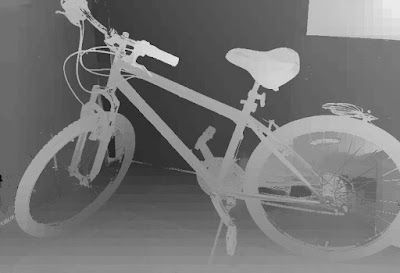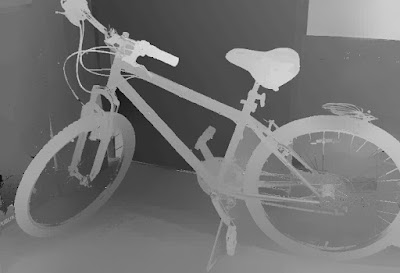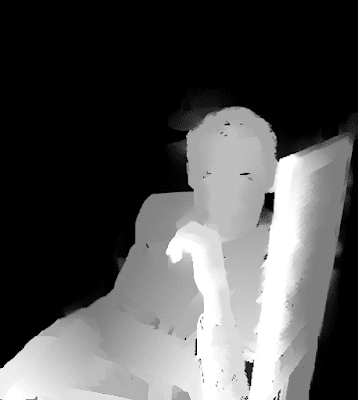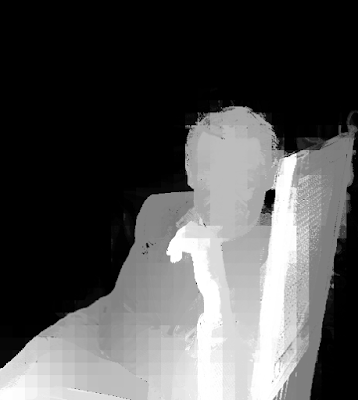Sunday, December 20, 2015

3D Photos - Bas-relief of a guy with an axe

The stereo pair came from Photosculpt, a company that makes a software which takes a front image and a right image (off by twenty degrees) and produces a 3d texture out of it that can be used in 3d modeling (in programs like Blender).

First, the two images need to be properly rectified. I am gonna use Epipolar Rectification 9 (ER9) to rectify the images.

I am gonna use Depth Map Automatic Generator 5b (DMAG5b) because there's not a lot of depth in the scene, and therefore not a whole lot of occlusions. DMAG5b needs a min and max disparity which you can get by carefully reading the output of the epipolar rectifier ER9. The output of ER9 gives min disparity = -12 and max disparity = 102. Those need to be multiplied by -1. So, for DMAG5b, min disparity = -102 and max disparity = 12.Depth map generated by DMAG5b using alpha = 0.9, truncation (color) value = 7.0, truncation (gradient) value = 2.0.

As advertised, there is not a lot of occlusions, which justifies the use of DMAG5b. If the 3d scene had more depth, DMAG5b would probably not be the best choice as it would "fatten" object boundaries.

3D Photos - Old stone wall

The stereo pair came from Photosculpt, a company that makes a software which takes a front image and a right image (off by twenty degrees) and produces a 3d texture out of it that can be used in 3d modeling (in programs like Blender).

As always, before even dreaming of getting a depth map, the two images need to be rectified so that the epipolar lines are horizontal, a requirement for most automatic depth map generators. I am gonna use Epipolar Rectification 9 (ER9) to rectify the stereo pair.

This kinda of stereo pairs where there's not much depth and occlusions is perfect for Depth Map Automatic Generator 5b (DMAG5b), a window-based depth map generator that's not edge-aware. If you look closely at the output given by the epipolar rectifier ER9, you can see that it gives a min and max disparities, in this case, -202 and -25. Well, these min and max disparities can be used as input to DMAG5b (and all the other automatic depth map generators) but they have to be reversed, in other words, the min disparity is 25 and the max disparity is 202.Depth map generated by DMAG5b using radius = 17, alpha = 0.9, truncation value (color) = 7.0, truncation value (gradient) = 2.0.

As you can see, there's not a whole lot of occluded pixels (in black).

These could be smoothed out by an edge preserving like Edge Preserving Smoothing 9 (EPS9) or by a Gaussian filter.

Of course, one could have also used our favorite general purpose automatic depth map generator, Depth Map Automatic Generator 7 (DMAG7)Depth map produced by DMAG7 using spatial sample rate = 16, color sample rate = 16, radius = 12, lambda = 0.01.Depth map produced by DMAG7 using spatial sample rate = 16, color sample rate = 16, radius = 12, lambda = 0.1.Depth map produced by DMAG7 using spatial sample rate = 16, color sample rate = 16, radius = 12, lambda = 1.Depth map produced by DMAG7 using spatial sample rate = 32, color sample rate = 16, radius = 12, lambda = 0.1.Depth map produced by DMAG7 using spatial sample rate = 16, color sample rate = 32, radius = 12, lambda = 0.1.

These depth maps (obtained by DMAG7) could probably be smoothed by Edge Preserving Smoothing 7 (EPS7), the so-called recursive domain filter.

Friday, December 18, 2015

3D Photos - Middlebury's bicycle

This is to illustrate the use of Depth Map Automatic Generator 9b (DMAG9b) (implementation of Jon Barron's Fast Bilateral Solver) to improve the quality of a given depth map (obtained by whatever means).Left image of Bicycle2 stereo pair. This is the quarter version of the full size image.

Here, we are gonna use Depth Map Automatic Generator 2 (DMAG2) to get an initial depth map.

Note that this is a rather small radius. Now, we want DMAG9b to improve that depth map.Depth map obtained by DMAG9b using 4 for the spatial bandwidth, 16 for the color bandwidth, and 0.5 for lambda.Depth map obtained by DMAG9b using 8 for the spatial bandwidth, 16 for the color bandwidth, and 0.5 for lambda.Depth map obtained by DMAG9b using 16 for the spatial bandwidth, 16 for the color bandwidth, and 0.5 for lambda.Depth map obtained by DMAG9b using 8 for the spatial bandwidth, 16 for the color bandwidth, and 5.0 for lambda.Depth map obtained by DMAG9b using 8 for the spatial bandwidth, 16 for the color bandwidth, and 50.0 for lambda.

Well, you get the idea. You can also play with the color bandwidth aka the range (color) sample rate. Note that the spatial bandwidth is also known as the spatial sample rate. DMAG9b can drastically improve the depth map quality, especially at object boundaries.

Of course, one could also have used Depth Map Automatic Generator 7 (DMAG7) (implementation of Jon Barron's Fast Bilateral Space Stereo) right from the start.Depth map obtained by DMAG7 using 16 for the spatial sample rate, 16 for the color sample rate, 2 for the radius, and 0.1 for lambda.

Again, note the low radius used.Depth map obtained by DMAG7 using 16 for the spatial sample rate, 16 for the color sample rate, 2 for the radius, and 0.01 for lambda.

You can check how this stacks up against competing automatic depth map generators at Middlebury Stereo Evaluation - Version 3.

Wednesday, December 16, 2015

2D to 3D Conversion - Nathan Fillion as Firefly's Malcolm Reynolds

This 2d to 3d image conversion highlights the use of Depth Map Automatic Generator 9 (DMAG9) to "densify" a sparse depth map while being edge-aware.

In my dmag9_input.txt, I used:
Spatial sample rate = 8
Range (color) sample rate = 16
Lambda = 0.5
Hash table size = 10000
Number of PCG (Preconditioned Conjugate Gradient) iterations = 1000
Scale parameter of Geman-McClure function = 1.41
Number of IRLS (Iteratively Reweighted Least Squares) iterations = 1

In general, if DMAG9 leaves out black pixels in the depth map, you can either slightly blur the reference image (Gaussian blur is fine) or increase the spatial sample rate.

2D to 3D Conversion - Harrison Ford in Blade Runner

I started with a sparse depth map scribbled in Gimp on top of the "Blade Runner" movie still:

Then, I called upon Depth Map Automatic Generator 4 (DMAG4) to generate the dense depth map:

In DMAG4, I used 5000 for the number of iterations and 1 for the number of scales. In other words, it's plain Random Walks.

Finally, I went on WiggleMaker to create a 3d wiggle animated gif:

Tuesday, December 15, 2015

2D to 3D Conversion - Cary Grant

This is to compare Depth Map Automatic Generator 4 (DMAG4) which is based upon "Image segmentation using Scale-Space Random Walks" by R. Rzeszutek et al. and Depth Map Automatic Generator 9 (DMAG9) which is based upon "The Fast Bilateral Solver" by J. Barron et al. It's in the context of 2d to 3d image conversion, in other words, edge-aware densification (propagation) of a sparse depth map.Dense depth map obtained by DMAG4 using max number of iterations = 1000, number of scales = 1, level of graph connection within a scale = 2, and level of graph connection across scales= 1.

Because there are some areas that are totally black when they should not be, it would be a good idea to crank up the number of iterations.Dense depth map obtained by DMAG4 using max number of iterations = 2000, number of scales = 1, level of graph connection within a scale = 2, and level of graph connection across scales= 1.Dense depth map obtained by DMAG4 using max number of iterations = 3000, number of scales = 1, level of graph connection within a scale = 2, and level of graph connection across scales= 1.Dense depth map obtained by DMAG4 using max number of iterations = 4000, number of scales = 1, level of graph connection within a scale = 2, and level of graph connection across scales= 1.Dense depth map obtained by DMAG4 using max number of iterations = 5000, number of scales = 1, level of graph connection within a scale = 2, and level of graph connection across scales= 1.

Clearly, things get better as the number of iterations increases. Now, let's bring the number of iterations back down and increase the number of scales.Dense depth map obtained by DMAG4 using max number of iterations = 1000, number of scales = 2, level of graph connection within a scale = 2, and level of graph connection across scales= 1.Dense depth map obtained by DMAG4 using max number of iterations = 1000, number of scales = 3, level of graph connection within a scale = 2, and level of graph connection across scales= 1.

Clearly, as the number of scales increases, DMAG4 is less susceptible to noise and creates smoother gradations in the presence of noise/texture (back of the chair). Unfortunately, some object boundaries become blurrier (Cary's hair bleeds into the background).

As a finale, let's use a high number of iterations and high number of scales.Dense depth map obtained by DMAG4 using max number of iterations = 5000, number of scales = 3, level of graph connection within a scale = 2, and level of graph connection across scales= 1.

Not much a difference with the previous depth map, which means it's probably quite safe to reduce the number of iterations when increasing the number of scales.

Now, let's see what the challenger, DMAG9, can do.Dense depth map obtained by DMAG9 using Spatial sample rate = 4, Range (color) sample rate = 16, Lambda = 0.5, Hash table size = 10000, Number of PCG (Preconditioned Conjugate Gradient) iterations = 1000, Scale parameter of Geman-McClure function = 1.41, and Number of IRLS (Iteratively Reweighted Least Squares) iterations = 1.

Let's increase the range (color) sample rate to 32 in order to reduce the number of colors to (256/32)^3 = 512. This should reduce the number of un-propagated black pixels.Dense depth map obtained by DMAG9 using Spatial sample rate = 4, Range (color) sample rate = 32, Lambda = 0.5, Hash table size = 10000, Number of PCG (Preconditioned Conjugate Gradient) iterations = 1000, Scale parameter of Geman-McClure function = 1.41, and Number of IRLS (Iteratively Reweighted Least Squares) iterations = 1.

Some smoothness can be gained by increasing lambda.Dense depth map obtained by DMAG9 using Spatial sample rate = 4, Range (color) sample rate = 32, Lambda = 100, Hash table size = 10000, Number of PCG (Preconditioned Conjugate Gradient) iterations = 1000, Scale parameter of Geman-McClure function = 1.41, and Number of IRLS (Iteratively Reweighted Least Squares) iterations = 1.

To improve the behavior of DMAG9, it's usually a good idea to blur the reference image.Blurred version of the reference image. I used Gimp's Gaussian Blur (bandwidth = 5 pixels in both directions).

Alright, let's do another round of DMAG9 passes on this blurred reference image.Dense depth map obtained by DMAG9 using Spatial sample rate = 4, Range (color) sample rate = 16, Lambda = 0.5, Hash table size = 10000, Number of PCG (Preconditioned Conjugate Gradient) iterations = 1000, Scale parameter of Geman-McClure function = 1.41, and Number of IRLS (Iteratively Reweighted Least Squares) iterations = 1.Dense depth map obtained by DMAG9 using Spatial sample rate = 4, Range (color) sample rate = 32, Lambda = 0.5, Hash table size = 10000, Number of PCG (Preconditioned Conjugate Gradient) iterations = 1000, Scale parameter of Geman-McClure function = 1.41, and Number of IRLS (Iteratively Reweighted Least Squares) iterations = 1.Dense depth map obtained by DMAG9 using Spatial sample rate = 4, Range (color) sample rate = 32, Lambda = 100, Hash table size = 10000, Number of PCG (Preconditioned Conjugate Gradient) iterations = 1000, Scale parameter of Geman-McClure function = 1.41, and Number of IRLS (Iteratively Reweighted Least Squares) iterations = 1.

At this point in time, it looks like DMAG4 (Scale Space Random Walks) has the edge over DMAG9 (Fast Bilateral Solver) in the context of 2d to 3d image conversion. As far as DMAG4 is concerned, I am not at all convinced that Scale Space Random Walks (number of scales > 1) is that much better than plain Random Walks (number of scales = 1) but it's there as an option.

Update (12/16/2015):

After publishing this blog post, I realized that I had not played around with the spatial sample rate. So, let's go back to our "base" experiment before increasing the spatial sample rate in an effort to get rid of those "black" pixels. Note that it's a slightly different sparse depth map (modified around Cary's magnificent hair).Dense depth map obtained by DMAG9 using Spatial sample rate = 4, Range (color) sample rate = 16, Lambda = 0.5, Hash table size = 10000, Number of PCG (Preconditioned Conjugate Gradient) iterations = 1000, Scale parameter of Geman-McClure function = 1.41, and Number of IRLS (Iteratively Reweighted Least Squares) iterations = 1.Dense depth map obtained by DMAG9 using Spatial sample rate = 8, Range (color) sample rate = 16, Lambda = 0.5, Hash table size = 10000, Number of PCG (Preconditioned Conjugate Gradient) iterations = 1000, Scale parameter of Geman-McClure function = 1.41, and Number of IRLS (Iteratively Reweighted Least Squares) iterations = 1.Dense depth map obtained by DMAG9 using Spatial sample rate = 16, Range (color) sample rate = 16, Lambda = 0.5, Hash table size = 10000, Number of PCG (Preconditioned Conjugate Gradient) iterations = 1000, Scale parameter of Geman-McClure function = 1.41, and Number of IRLS (Iteratively Reweighted Least Squares) iterations = 1.

If I had the patience, I would then proceed to apply a bit of edge-aware smoothing, for instance, Edge Preserving Smoothing 7 (EPS7). In the end, DMAG9 appears to be doing as good a job as DMAG4 as long as you're using the right parameters.